Latest Banking jobs   »

# Quantitative Aptitude Quiz For Bank Foundation 2023 -25th May

Directions (1-5): The following questions are accompanied by two statements (I) and statement (II). You have to determine which statements(s) is/are sufficient/necessary to answer the questions given below.
(a) Statement (I) alone is sufficient to answer the question but statement (II) alone is not sufficient to answer the question.
(b) Statement (II) alone is sufficient to answer the question but statement (I) alone is not sufficient to answer the question.
(c) Both the statements taken together are necessary to answer the question, but neither of the statements alone is sufficient to answer the question.
(d) Either statement (I) or statement (II) by itself is sufficient to answer the question.
(e) Statements (I) and (II) taken together are not sufficient to answer the question.

Q1. Curved surface area of a canonical box is 136π cm². Find the volume of the canonical box?
I. Slant height of the canonical Box is 17 cm.
II. Area of the base of canonical box is 64π cm².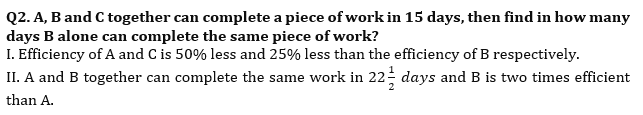Q3. Speed of train-A is 20% more than the speed of train-B and length of train-A is 120m more than length of train-B. find the Speed of train-A?
I. If train-A crosses a pole in 18 sec and train-B crosses the same pole in 72/5 sec.
II. If both trains passed each other in 180 sec while moving in opposite direction.

Q4. A shopkeeper marks price of an article 40% above its cost price and gives a discount of r% on it. Find r?
I. If cost price of the article is Rs. 2500.
II. If selling price of the article is Rs. 300 more than the cost price of the article.

Q5. A bag contains x red balls, y blue balls and 10 green balls. Find the total number of red and blue balls in the bag?
I. If one ball is drawn from bag and probability of being that ball Red is 5/22.
II. If one ball is drawn from bag and probability of being that ball Blue is 7/22.

Directions (6-10): In the following questions two quantities (Quantity I and Quantity II) are given for each question. Compare the numeric value of both the quantities and answers accordingly.

Q6. Quantity I – A boat can cover a certain distance in 50 hours and 70 hours in downstream and upstream respectively. Time taken by boat to cover same distance in still water.
Quantity II – Veer and Aayush together can complete a work in 240/7 hours and Aayush alone can complete the same work in 80 hours. Time taken by Veer to complete same work alone.
(a) Quantity I > Quantity II
(b) Quantity I < Quantity II
(c) Quantity I ≥ Quantity II
(d) Quantity I ≤ Quantity II
(e) Quantity I = Quantity II or no relation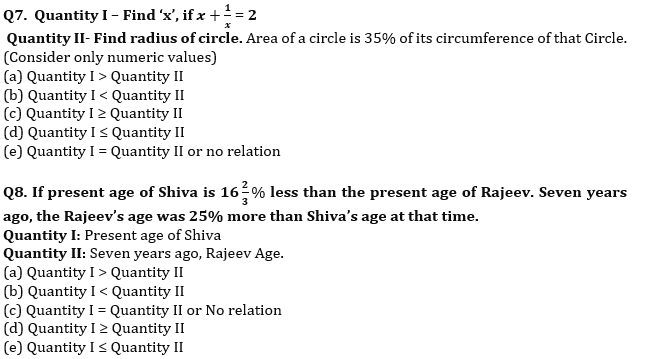Q9. The Perimeter of an isosceles right-angle triangle is (16+8√2) cm.
Quantity I : Find out the radius of circle which is inscribed in the triangle.
Quantity II : Find out the value of 75% of the radius of circumcircle of the triangle.
(a) Quantity I > Quantity II
(b) Quantity II > Quantity I
(c) Quantity I ≥ Quantity II
(d) Quantity II ≥ Quantity I
(e) Quantity I = Quantity II or relation can’t be established

Q10. A container contains 60 liters mixture of alcohol and water.
Quantity I. Initial quantity of alcohol. if 6 liters water is added in the container then ratio of alcohol to water becomes 6:5.
Quantity II: 60% more than initial quantity of water. if 15 liters alcohol is added in the container then ratio of alcohol to water becomes 8:7.
(a) Quantity I > Quantity II
(b) Quantity I < Quantity II
(c) Quantity I ≥ Quantity II
(d) Quantity I ≤ Quantity II
(e) Quantity I = Quantity II or no relation

Solutions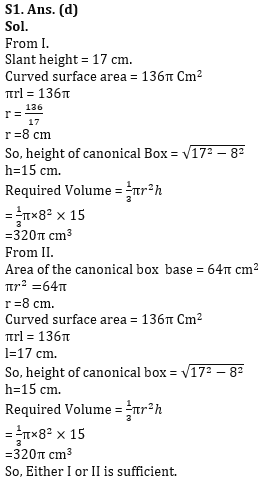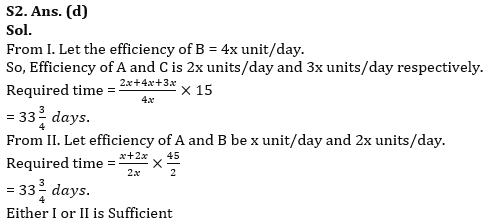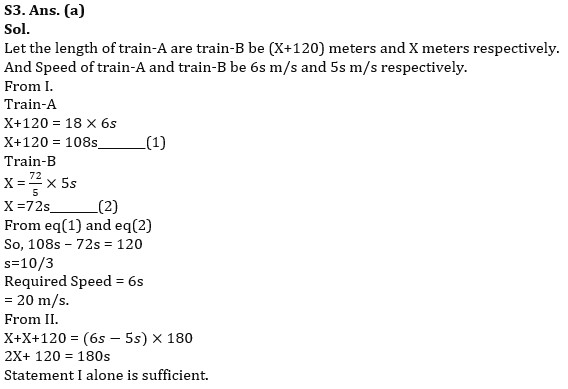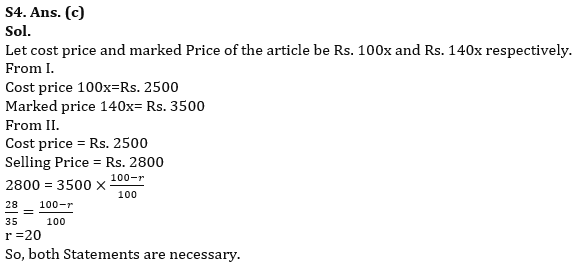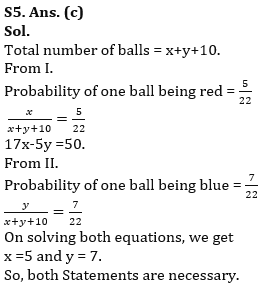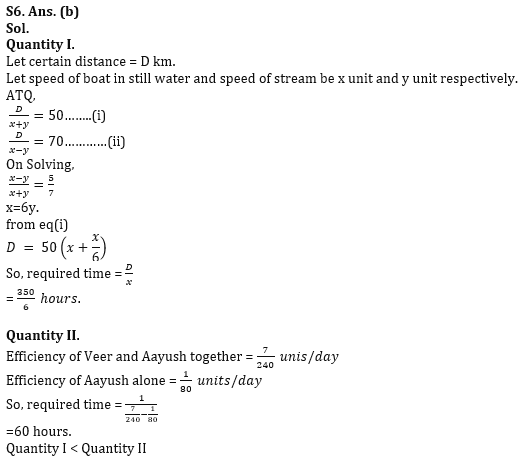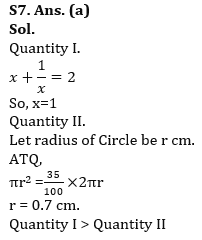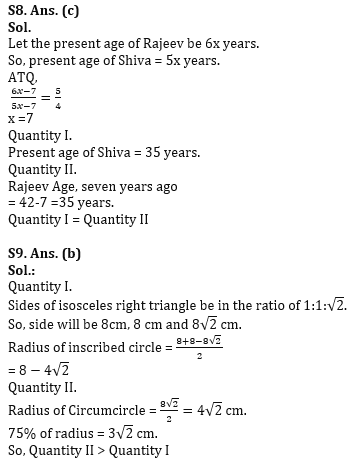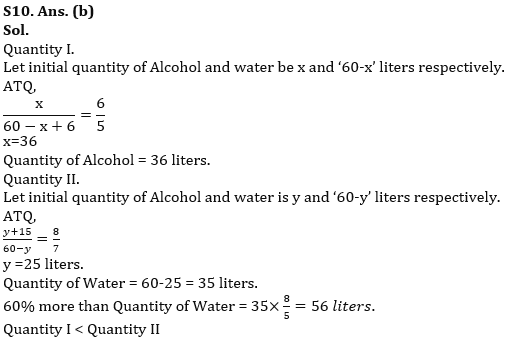.

## FAQs

### What is the selection process of the Bank Clerk?

The selection process of the Bank Clerk is Prelims & Mains.

#### Congratulations!Union Budget 2023-24: Free PDF